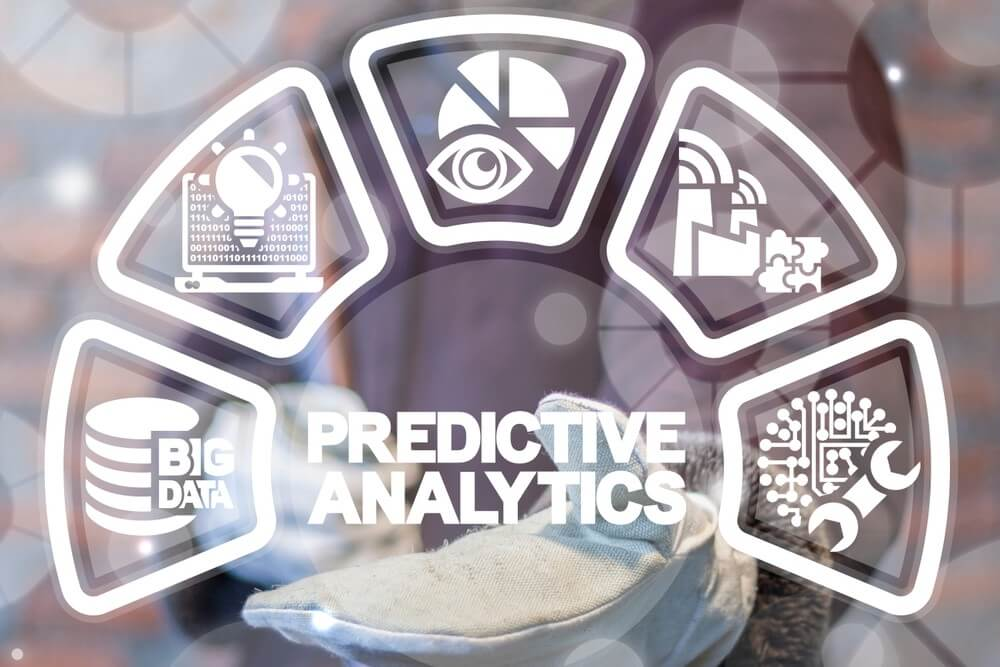AI / Machine Learning
-
September 4, 2019

# From Summarization to Generalization and PredictionData predictions provide probabilities of future outcomes by mining and analyzing existing data, also called training data. Effective prediction is a mix of engineering, statistics, and intuition. Summarization can help by shaping this intuition. In the generalization phase, we test our training data against new data, called test data, to calculate if our model is good enough to be used in real life. These two processes simplify large multidimensional datasets, so machine learning predictions can be applied to them. This article describes how summarization leads to generalization and then prediction through a real estate example.

These are basic definitions:

Summarization. Meaningful information is extracted from large sets of multidimensional data. This process can be time-consuming because big datasets often contain many different types of data.

Generalization. Summarized datasets are mined for only the data that’s relevant to a specific task.

### Real estate example

A real-life example is the data real estate companies collect on their properties. Let’s say that we have a list of 1001 apartments sold in New York City in March. For each property, there are many variables like these features:

• Square feet
• Number of bedrooms
• Number of bathrooms
• Neighborhood
• Final sale house

The first concept we’ll talk about is “feature. A feature is a piece of information about one of the examples in our dataset. In this case, we have 1001 apartments, and each one has six features.

### Summarizing real estate data

To understand our data a bit more, we might ask these questions:

• What’s the average selling price?
• What’s the median list price? The median is the price in the middle of a sorted list. An example is the 501st value in a list of 1000. We use medians to reduce the impact of extreme cases.
• What’s the square deviation in the area values? We can find that answer with this formula:

where:

• An is the area for sample n.
• Ā is the sample average of square footage.

This result is called the standard deviation. It’s defined as the square root of the average value of squared deviations. The square deviation is the squared value of the difference between each value and the sample average. By squaring this value, we really say that we’re only interested in the magnitude of the deviation, not the sign. And larger deviations will contribute more to this value.

All these calculations are various forms of summaries of what this specific real estate data tells us.

### Predictive analysis of pricing

In the real estate business, we probably want to know in advance what prices we can get for the property we sell. To get answers that are a bit more biased toward prediction, we might ask these questions:

• Which neighborhoods are associated with higher prices? This question highlights the relationship between two features in our dataset: neighborhood and price. To get the answer, we can compute the average sale price for each neighborhood and sort the results. Then we see how neighborhoods relate to average final sale prices.
• What’s the average delta, or difference between numbers, of the asking and selling prices?
• What’s the price per square feet?
• How do number of square feet and number of bedrooms relate to pricing?

When we look into more and more associations, the size of our dataset starts to limit us. And as we add more and more features into our relationships, we get sparser data. An example is making calculations on four-bedroom apartments larger than 12.000 square feet in the Chelsea neighborhood. The bottom line is that the more complex the question, the more pressure there is to have a larger dataset.

### Summarization

The summarization process captures patterns in our datasets. When summarizing, we don’t try to extrapolate beyond the data. We only want real representations of the patterns in existing data.

These examples are more types of summarization:

• Data visualizations like charts, plots, and histograms.
• Clustering and grouping similar data points. The goal of clusterization is to uncover patterns in data, without any prior guidance or any specified outcomes. This algorithm is a technique of unsupervised learning.

The main goal of summarization is to get a better understanding of what we are dealing with, which will help us to make decisions later in the process of building machine learning algorithms.

### Prediction and generalization

To make predictions, we create a model of reality and then analyze past data to forecast the future. This type of analysis is called supervision. Prediction is a technique of supervised learning.

When we make predictions, we make statements that go beyond the characteristics or dimensions of the data we’re analyzing. Predictions step outside our existing dataset. In this process, we analyze the data to produce assumptions about the underlying principles of the domain. The process of using a dataset to reason about the world outside the data is called generalization.

### Data samples

In our example, the world outside our data is the broader real estate population. In this context, it could be all the apartments in New York City. Because it’s difficult to gather or analyze the enormous datasets of entire populations, we often analyze relevant samples to reason about a population.